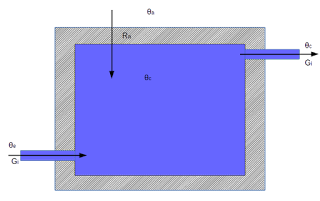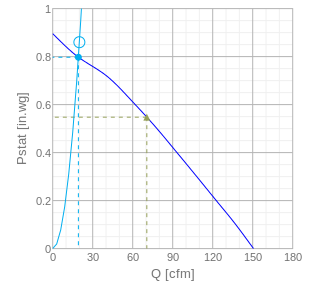Thermodynamic calculcations for cold roomSimplified thermodynamic model of cold room

I built a cold room in my basement by insulating the basement space under the stairs and bringing in cold air from the garage through 2" central vacuum tubing.

I have insulated all walls and the door with two inch foam board which should give it an R value of 7.5. The floor is not insulated, it is bare concrete which stays pretty cold.
The ambient temperature around the cold room is 22 Celcius; the furnace room is adjacent to the cold room and the furnace ducts have lots of air leaks (as most forced air systems seem to have).

I have measured temperatures in the garage and the cold room; now I'd like to figure out what the thermal resistance (or R value) of my cold room is.
First, I need to calculate how much air is coming into the cold room.  The air performance graphs of the FR199 show that the fan operates between 0 and .90 inches of water column static pressure; at 0 static pressure it delivers 137 CFM, at .90" static pressure it produces 0 CFM and the curve is almost linear.
I measured the length of vacuum pipe I used, which is a total of 36 feet of pipe with two 90 degree bends and three 45 degree bends. I plugged all the data into a static pressure calculator spreadsheet, trying to figure out the duct air flow. I entered several numbers in the Duct Airflow (CFM) box until I saw a friction loss number that fell on the fan's curve:Fantech FR100 flow rate versus static pressure
So the flow rate must be around 20 cubic feet per minute, or 0.009439 cubic meters per second (all my calculations below use SI or metric units because that is easier).

With the air input flow rate figured out, we can move on to the thermodynamic equation of the cold room in equilibrium. I have found  the cold room in thermodynamic equilibrium with garage air of 6 Celcius, cold store temperature of 15 Celcius and an ambient temperature of 22 Celcius.

The thermodynamic model shows garage air at temperature θe coming in a flow rate of Gi. The inside of the cold room is at temperature θc and the air exiting the cold room is at that same temperature, at the same flow rate Gi. Simplifying the cold room into an insulated box with thermal resistance Ra, the heat coming in from ambient temperature θa equal to (θa - θe)/Ra.

If this system is in equilibrium, the following equation applies, which lets us solve Ra:

Gi = flow rate of incoming (and outgoing) air
Ci = heat capacity of air
Θe = temperature of external air being pulled in
Θc = temperature of the cold room
Θa = ambient temperature of basement around the cold room
Ra = thermal resistance of the cold roomThe heat capacity of air at 6 degrees Celcius is 1.003 kJ/kg Kelvin. The density of air around 6 degrees Celcius is 1.2690 kg per cubic meter.

With air temperatures translated from Celcius to Kelvin (θe=279.15 K, θc=288.15 K and θa= 295.15 K), our formula then becomes:

Solving for Ra: 0.012014 kJ / K.s . -9 K + 7K/Ra  = 0 or 0.1081 kJ/s = 7 K/Ra

One Joule per second being a Watt, this calculates Ra as 64.8 Kelvin per kW.

To translate that to an R value, we need the total surface of the cold room. I measured that around 10.9 square meters.

The SI R value is then 64.8 K/kW . 10.9 m^2 = 0.71 K.m^2/W
To convert that to US R values, multiply by 5.687 = 4.0 sq ft . degree Fahrenheit . hr / Btu

So although all surfaces are insulated to R7, effectively the cold room has a thermal resistance of R4.

Well that was fun, don't you think?

UPDATE 10 Dec 2016

I measured the temperature of the cold air exiting inside the cold room when the outside air was -10.7C: the air had heated up on its way through the pipe to +5.7C. So there is probably nothing wrong with the cold room insulation, but the un-insulated pipe is causing massive loss of "coldness".